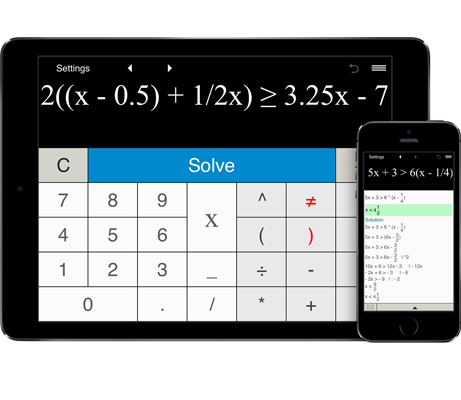# Linear inequality calculator

Linear inequality calculator can handle a wide range of linear inequalities, including linear inequalities with fractions and parentheses.
Shows step-by-step solution.

## Linear inequality calculator with steps

This calculator solves linear inequalities in one variable, including linear inequalities involving fractions and parentheses. Just enter a linear inequality, click the Solve button and get the step-by-step solution.

You can easily solve one-step, two-step and multi-step linear inequalities.

Linear Inequality Calculator stores 25 last inequalities and lets you easily recall previous inequalities from the Calculation history using the arrows buttons.

To prevent accidental deletion of your inequality with the "C" button, Linear Inequality Calculator provides the "Undo" button.

Note:
Linear Inequality Calculator solves linear inequalities for x.

Linear Inequality Calculator does not solve linear inequalities with a variable in the denominator of a fraction.

Linear Inequality Calculator does not support linear inequalities with two variables.